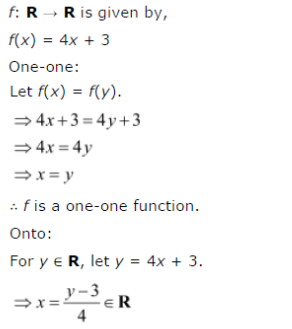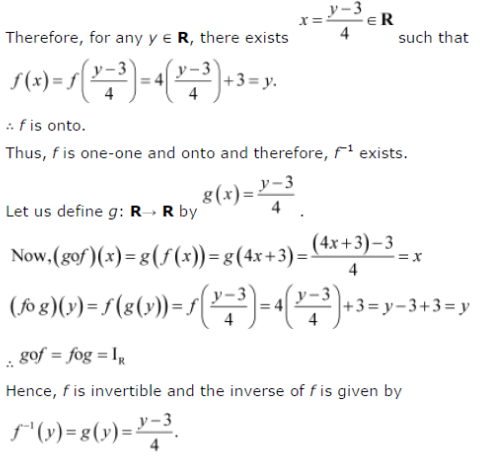# Consider f : R → R given by f(x) = 4x + 3. Show that f is invertible. Find the inverse of f

Consider f : R → R given by f(x) = 4x + 3. Show that f is invertible. Find the inverse of f .